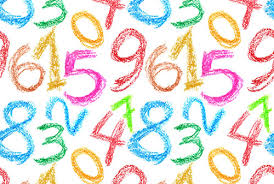# Easy Pace Learning

### Exercises

Write the numbers in words exercise

## How to complete the exercise on writing the numbers in words?

To complete the exercise write the number in words next to each number.

## Writing the number in words exercise

Write the correct word next to each number

64   = sixty-four

13 =

16 =

88 =

61 =

35 =

12 =

30 =

342 = three hundred and forty-two

768 =

234 =

633 =

999 =

## Lessons and exercises that are relatedTo view any of the lessons below click on link.

Matching numbers exercise

Ordinal numbers ranking English lesson

English Numbers 1 to 100 learning cardinal numbers

## Print the exercises

To print the exercise on matching the numbers and words right click on a white space and choose print. You can click on the printer icon just below and to the right of the contact us menu button at the top of the page or copy and paste the part of the exercise you want onto a word document and then print onto some paper.

## Dictionary and how to use dictionaries

Click on the following link for the Online English dictionary - English lesson